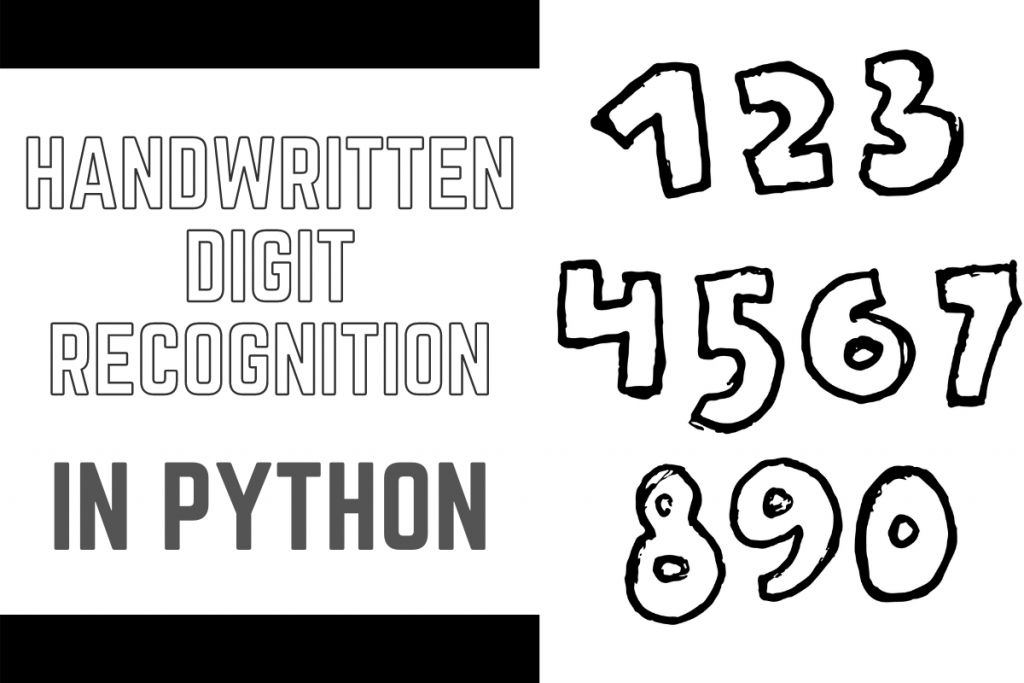# Handwritten Digit Recognition in PythonHello learner! Today in this tutorial, we will learn how to recognize handwritten digits from the MNIST dataset already available in sklearn datasets. To recognize digits we will make use of the Convolutional Neural Networks (CNN).

Let’s first start by understanding what CNN is.

## What is Convolutional Neural Network?

CNN is one of the most important neural network models for computing tasks based on multi-layered perceptron. These models perform particularly well for the processing of images. For instance, recognition of handwriting. Handwriting Recognition is one of neural networks’ most basic and excellent uses. CNN model is trained in multiple layers to make the correct predictions

### Convolutional Neural Network use cases

CNN is playing an important role in sectors like image processing. It holds a powerful impact on detections and predictions. It’s even used in nanotechnologies like manufacturing semiconductors. Here, it’s used to detect faults in the material. If CNN is used with Keras or Tensorflow, it gives the highest accuracy as compared to various classification algorithms. CNN along with back-propagation architecture results in the highest accuracy with the MNIST dataset as compared to any other datasets. New applications are developing using CNN day by day through research. In Germany, a traffic sign recognition model using CNN is suggested.

The data set that we are going to use contains around 60,000 training images and 10000 testing images. We then split the data into training and testing datasets respectively.

The `x_train` and `x_test` contains the pixel codes for images while `y_test` and `y_train` contains labels from `0–9` which represents the numbers as the digits can vary from 0 to 9.

Now we need to check if the shape of the dataset is ready to use in the CNN model or not. The size of data is observed as (60000,28,28) which implies 60000 images of size 28×28 pixel each.

But in order to use Keras API we need a 4-dimensional array dataset hence we need to convert the 3-D data into 4-D dataset.

```import tensorflow as tf
(x_train, y_train), (x_test, y_test) = tf.keras.datasets.mnist.load_data()

x_train = x_train.reshape(x_train.shape, 28, 28, 1)
x_test = x_test.reshape(x_test.shape, 28, 28, 1)
input_shape = (28, 28, 1)
```

The next step is normalizing the data, for which first the data is convered to float and then it is divided by 255 (maximum RGB code – minimum RGB code).

```x_train = x_train.astype('float32')
x_test = x_test.astype('float32')

x_train /= 255
x_test /= 255
```

## Building the Model

In this tutorial, use will make use of the Keras API to build the model and in order to do that we will be importing the Sequential Model from Keras and adding multiple layers which are listed below:

1. Conv2D
2. MaxPooling
3. Flatten
4. Dropout
5. Dense

Dropout layers are responsible to fight with the overfitting and the Flatten layers flatten the 2D arrays to 1D arrays.

```from tensorflow.keras.models import Sequential
from tensorflow.keras.layers import Dense, Conv2D, Dropout, Flatten, MaxPooling2D
model = Sequential()
```

## Compiling and fitting the Model

So now that we have created an non-optimized empty CNN. We then set an optimizer with a given loss function which makes use of a metric and the model is fit by using the train dataset created. The ADAM optimizer outperforms other similar optimizers.

```model.compile(optimizer='adam', loss='sparse_categorical_crossentropy', metrics=['accuracy'])
model.fit(x=x_train,y=y_train, epochs=10)
```

The results of the training process are as follows.

On evaluation of the model using the `evaluate` function, we observe an accuracy of 98.4%.

## Visualizing the results

Our final step is the visualize the results of the trained model and plot them with the help of `subplots`. The code and output for the same is shown below. We can see that the results are pretty accurate.

```import matplotlib.pyplot as plt
plt.style.use('seaborn')

plt.figure(figsize=(10,10))
plt.subplot(4,4,1)
image_index = 2853
predict = x_test[image_index].reshape(28,28)
pred = model.predict(x_test[image_index].reshape(1, 28, 28, 1))
plt.imshow(x_test[image_index].reshape(28, 28),cmap='Greys')
plt.title("Predicted Label: "+str(pred.argmax()))

plt.subplot(4,4,2)
image_index = 2000
predict = x_test[image_index].reshape(28,28)
pred = model.predict(x_test[image_index].reshape(1, 28, 28, 1))
plt.imshow(x_test[image_index].reshape(28, 28),cmap='Greys')
plt.title("Predicted Label: "+str(pred.argmax()))

plt.subplot(4,4,3)
image_index = 1500
predict = x_test[image_index].reshape(28,28)
pred = model.predict(x_test[image_index].reshape(1, 28, 28, 1))
plt.imshow(x_test[image_index].reshape(28, 28),cmap='Greys')
plt.title("Predicted Label: "+str(pred.argmax()))

plt.subplot(4,4,4)
image_index = 1345
predict = x_test[image_index].reshape(28,28)
pred = model.predict(x_test[image_index].reshape(1, 28, 28, 1))
plt.imshow(x_test[image_index].reshape(28, 28),cmap='Greys')
plt.title("Predicted Label: "+str(pred.argmax()))
```